# Atomic Structure IPage 2

#### WATCH ALL SLIDES

Light is a wave…right?

Einstein’s interpretation of the photoelectric effect (1905) was that light is quantized in packets of set energy called photons. (He won the Nobel Prize for this.)

This meant that light had characteristics of particles!

Slide 13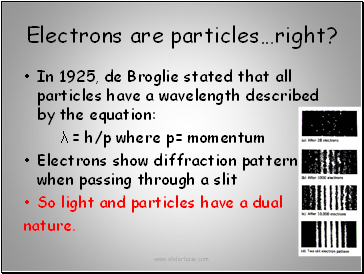## Electrons are particles…right?

In 1925, de Broglie stated that all particles have a wavelength described by the equation:

λ = h/p where p= momentum

Electrons show diffraction pattern when passing through a slit

So light and particles have a dual

nature.

Slide 14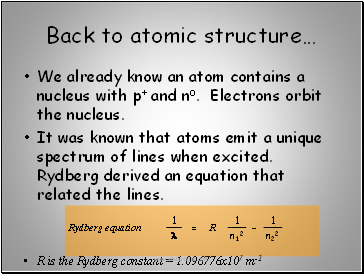## Back to atomic structure…

We already know an atom contains a nucleus with p+ and no. Electrons orbit the nucleus.

It was known that atoms emit a unique spectrum of lines when excited. Rydberg derived an equation that related the lines.

R is the Rydberg constant = 1.096776x107 m-1

Slide 15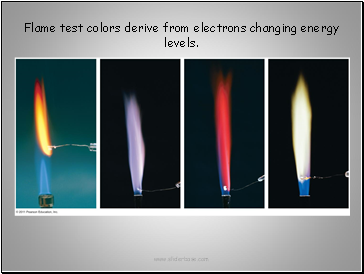Flame test colors derive from electrons changing energy levels.

Slide 16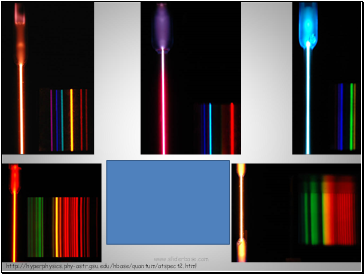http://hyperphysics.phy-astr.gsu.edu/hbase/quantum/atspect2.html

Atomic emission spectra

Clockwise from lower left:

neon, helium, hydrogen,

mercury, nitrogen

Slide 17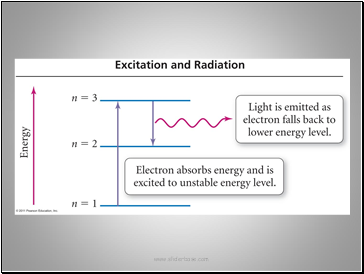Slide 18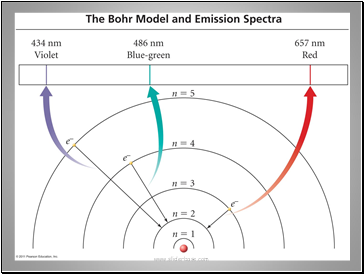Slide 19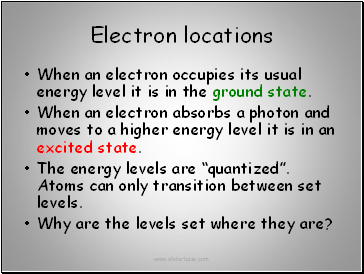## Electron locations

When an electron occupies its usual energy level it is in the ground state.

When an electron absorbs a photon and moves to a higher energy level it is in an excited state.

The energy levels are “quantized”. Atoms can only transition between set levels.

Why are the levels set where they are?

Slide 20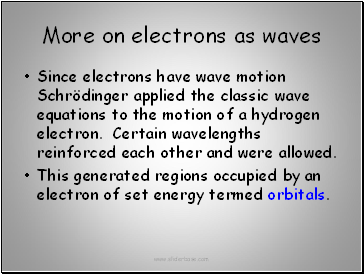## More on electrons as waves

Since electrons have wave motion Schrödinger applied the classic wave equations to the motion of a hydrogen electron. Certain wavelengths reinforced each other and were allowed.

This generated regions occupied by an electron of set energy termed orbitals.

Slide 21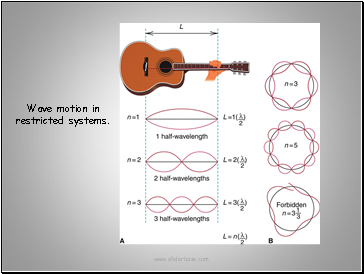Wave motion in restricted systems.

Slide 22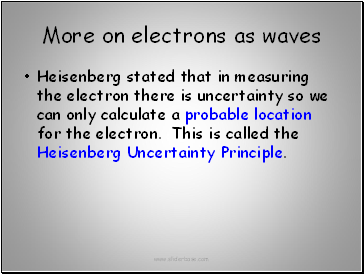More on electrons as waves

Heisenberg stated that in measuring the electron there is uncertainty so we can only calculate a probable location for the electron. This is called the Heisenberg Uncertainty Principle.

Go to page:
1  2  3